# WishXUI drawline/rectangle straight line as non antialised

#### sorex

##### Expert
Hello,

Can we have an option or fix to be able to draw straight lines as intended to be.

The current issues I see are...

- roundings due to anti aliasing
- alpha changes due to anti aliasing causing color changes on overlapping
- a 1 pixel line is always 2 pixels due to the anti aliasing
- you need to make your canvas 2 pixels bigger in width in height or you'll get thinner lines at the edges due to the 2 pixel lining (that why you get these 1+ in the code lines below)

anti aliasing should not be active for rectangle and straight non-angled lines.code used for this is:

B4X:
``````Dim c As B4XCanvas
Dim p As Pane=xui.CreatePanel("grid")
c.Initialize(p)

For x=0 To gridSize-1
c.Drawline(1+x*cellsize,1,1+x*cellsize,1+gridSize*cellsize,col,1)
Next

For y=0 To gridSize-1
c.Drawline(1,1+y*cellsize,1+gridSize*cellsize,1+y*cellsize,col,1)
Next

Dim r As B4XRect
r.Initialize(1,1,gridSize*cellsize+1,gridSize*cellsize+1)
c.DrawRect(r,col,False,1)``````

Last edited:

#### Erel

Staff member
Not the most elegant solution but you can use DrawRect to draw thin lines. DrawRect is not anti-aliased as the lines are always straight.

Making DrawLine treat differently straight lines will have negative side effects in other cases.

#### sorex

##### Expert
I forgot to mention that I tried drawrectangle aswell. The result was the same.

I just tried again to be sure with the code below and the image is similar.

B4X:
``````For x=0 To gridSize-1
Dim r As B4XRect
r.Initialize(1+x*cellsize,1,1+x*cellsize,1+gridSize*cellsize)
c.DrawRect(r,col,False,1)
Next

For y=0 To gridSize-1
Dim r As B4XRect         r.Initialize(1,1+y*cellsize,1+gridSize*cellsize,1+y*cellsize)
c.DrawRect(r,col,False,1)
Next

Dim r As B4XRect    r.Initialize(1,1,gridSize*cellsize+1,gridSize*cellsize+1)
c.DrawRect(r,col,False,1)``````

and the result zoomed in with 1 pixel grid enabled...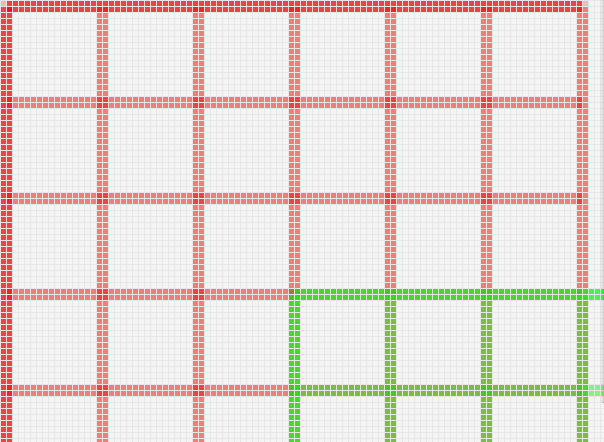#### sorex

##### Expert
here's the code seperated from the project

#### Attachments

• lines.zip
1.1 KB · Views: 104

#### sorex

##### Expert
so bitmapcreator is the only way to get the fine line as seen in the first square of your example?

I tried to use it but I also get the blurred 2px line.

I'll have a look at your file to see how you did it.

#### sorex

##### Expert
I think I know what's wrong...

The bitmap creator method is the only one that work but with a big BUT.

when using the following code:

B4X:
``````    r.Initialize(10,10,50,50) 'big red square
bc.DrawRect(r,col,False,1)

r.Initialize(60,50,70,50) ' red line
bc.DrawRect(r,col,False,1)

r.Initialize(80,50,90,51) ' blue line
bc.DrawRect(r,xui.Color_Blue,False,1)``````

you get this result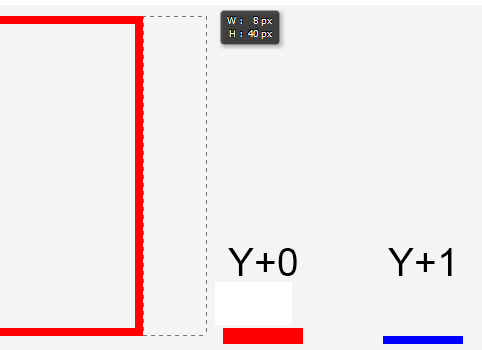1. the rectangle seems correct but to my logic it's 1 pixel too short. it goes from 10-49 and not 10-50.
so it draws exclusive the given value 50 and draws TILL 50 not TO 50.

2. when using a top & bottom that is equal (Y+0) the routine seems to draw an extra line on top. seems like some bottom - top lenght math that went wrong
so it appears that it start at Y:49 and ends at Y:50 while it is probably the other way around

3. when setting the bottom as top+1 (Y+1) it draws a single pixel line at Y=50. same math as step 2 solves it here ( len = (bottom-1)-top ?)

#### emexes

##### Expert
1. the rectangle seems correct but to my logic it's 1 pixel too short. it goes from 10-49 and not 10-50.
Yes, but on the other hand, how wide are you expecting the rectangle to be on the screen? 40 pixels, or 41?

To use a smaller example: how wide would you expect r.Initialize(0, 0, 3, 3) to be? What math did you use to work that out? If the resolution of the screen was doubled, so that everything on screen was twice-as-many pixels wider and taller, what would you expect the rectangle numbers to be now? How would you work that out? And what about if the resolution was tripled?

It is the same as with string indexing - slightly mindwarping at first, eg "not including the last character" but once over that conceptual hump, it does make the calculations nice, ie the length of the substring = the distance between the two indexes.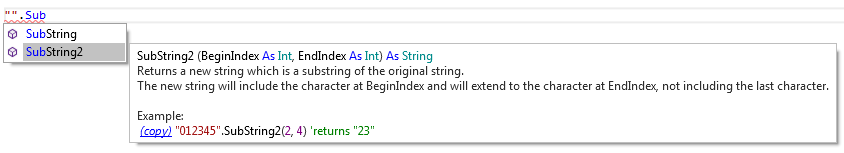2. when using a top & bottom that is equal (Y+0) the routine seems to draw an extra line on top. seems like some bottom - top length math that went wrong so it appears that it start at Y:49 and ends at Y:50 while it is probably the other way around
Your two examples are certainly a good example of the mindwarping aspects of pixel-vs-coordinate math.

EDIT: THE FOLLOWING WALKTHROUGH IS WRONG*, BECAUSE I DIDN'T ACCOUNT FOR STROKEWIDTH
* or at least, not as right as I would like it to be :-/

To be see the logic from another perspective, consider this:

What would you expect if you asked for a three-pixel-high rectangle eg (60, 50, 70, 53)? Height = Y2 - Y1 = 53 - 50 = 3. So far, so good.

The top line would be pixel line #50 and the bottom line would be pixel line #52, ie a rectangle that is three pixels high. We're still good.

Then ask for a two-pixel-high rectangle eg (60, 50, 70, 52). Height = Y2 - Y1 = 52 - 50 = 2. We're still good.

The top line would be pixel line #50 and the bottom line would shift up one to be pixel line #51, ie a rectangle that is two pixels high. We're still good.

Then ask for a one-pixel-high rectangle eg (60, 50, 70, 51). Height = Y2 - Y1 = 51 - 50 = 1. We're still good.

The top line would be pixel line #50 and the bottom line would shift up one to be pixel line #50, ie a rectangle that is one pixel high. We're still good again.

Now ask for a zero-pixel-high rectangle eg (60, 50, 70, 50). Height = Y2 - Y1 = 50 - 50 = 0. We're still good, although perhaps slightly nervous now.

The top line would still be pixel line #50 and the bottom line would shift up one to be pixel line #49, ie a rectangle that is... wtf?!?!... two pixels high. This is, I admit, not a result that anyone would expect, but it is entirely consistent with the pattern by which we got there.

I should have prefaced this post with advice to have an alcoholic beverage to hand ;-)

EDIT: AMEN TO THAT LAST LINE

Last edited:

#### emexes

##### Expert
Just had a small epiphany - perhaps if you offset your line's coordinates by 0.5, so that the coordinates are bang in the middle of the pixels, you might get an (antialiased) line that is 1 pixel wide on-screen.

At the moment, if you draw a one-pixel vertical line at say X = 7, then you could reasonably argue that the line's width (brush) is from X = 6.5 to X = 7.5, and thus pixel column #6 is half-filled (on its right side) and pixel column #7 is half-filled (on its left side).

Last edited:

#### emexes

##### Expert
Well, that worked better than I'd dared hope for (pessimists of the world, unite!)

Here is a set of one-pixel-wide (StrokeWidth = 1) lines, with fractional X offsets of: 0, 1/6, 2/6, 3/6 (ie 0.5), 4/6, 5/6 and 6/6.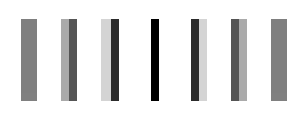I would have expected the top and bottom of each line to be antialiased too, since there is no fractional Y offset, but... probably best to quit while we're aheadB4X:
``````Dim X As Float
For X = 10 To 70 Step 5 + 1 / 6    'ie 5.1666...
c.Drawline(X, 10, X, 20, xu.Color_Black, 1)
Log("X = " & NumberFormat2(X, 1, 4, 4, False))
Next``````
B4X:
``````X = 10.0000
X = 15.1667
X = 20.3333
X = 25.5000
X = 30.6667
X = 35.8333
X = 41.0000
X = 46.1667
X = 51.3333
X = 56.5000
X = 61.6667
X = 66.8333``````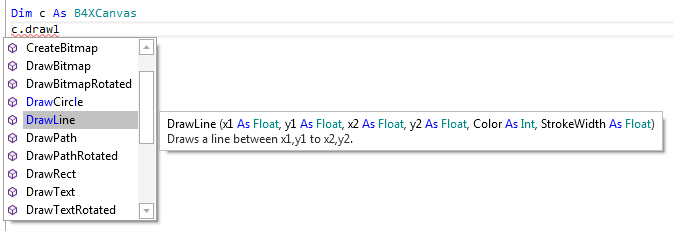Last edited:

#### emexes

##### Expert#### sorex

##### Expert
What would you expect if you asked for a three-pixel-high rectangle eg (60, 50, 70, 53)? Height = Y2 - Y1 = 53 - 50 = 3. So far, so good.

actually you're wrong here.

you write you expect a 3 pixel high rectangle but while 53-50 is indeed 3 you plot at 50,51,52,53 which are 4 pixels#### emexes

##### Expert
actually you're wrong here.
you write you expect a 3 pixel high rectangle but while 53-50 is indeed 3 you plot at 50,51,52,53 which are 4 pixelsYou might be right. And yet the results fit the anti-aliasing theory. Hmm. Hang on a second...

#### emexes

##### Expert
actually you're wrong here.

you write you expect a 3 pixel high rectangle but while 53-50 is indeed 3 you plot at 50,51,52,53 which are 4 pixelsRighto, worked it out - what we are actually plotting are pixels along the X coordinates X = 50 and X = 53, which are three pixels apart. But because StrokeWidth is one pixel wide, half of that stroke appears on each side of the lines, and thus the rectangle onscreen is one pixel (one stroke width) wider than 53 - 50.

nominally 3 pixel-wide boxes

(i) antialiased, and (ii) offset by half a pixelB4X:
``````Dim R As B4XRect
R.Initialize(120, 120, 123, 124)
Log("Width = " & R.Width)
Log("Height = " & R.Height)
c.DrawRect(R, xu. Color_Blue, False, 1)

'antialiasing workaround - offset coordinates by half a pixel:

Dim RR As B4XRect
RR.Initialize(140.5, 120.5, 143.5, 124.5)
Log("Width = " & RR.Width)
Log("Height = " & RR.Height)
c.DrawRect(RR, xu. Color_Blue, False, 1)``````

#### emexes

##### Expert
Same rectangles drawn with a StrokeWidth = 2.

The stroke on the left-hand rectangle now extends one pixel each side of the stroke lines, opacity 100% for both.

The stroke on the right-hand rectangle also extends one pixel each side, but from eg X = 139.5 to X = 141.5, thus lighting up:
- pixel column 139 with 50% opacity
- pixel column 140 with 100% opacity
- pixel column 141 with 50% opacity

I think the solution to the coordinates vs pixels confusion, is a display with infinite resolutionB4X:
``````Dim R As B4XRect
R.Initialize(120, 120, 123, 124)
c.drawRect(R, xu. Color_Blue, False, 2)

Dim RR As B4XRect
RR.Initialize(140.5, 120.5, 143.5, 124.5)
c.DrawRect(RR, xu. Color_Blue, False, 2)``````

#### emexes

##### Expert
actually you're wrong here.
The more I look at my post there, the wronger it gets with respect to rectangle sizes. Spewin! I'm tempted to delete it, but instead I'll add a notice at the top that it's wrong because I forgot about the stroke width taking up extra room.

#### sorex

##### Expert
no worries. I think it all comes to the point of interpretation.

if you take literally what is mentioned in the drawline description screenshot then you would think it would draw a line from 51 to 52 in our example.
as that is 'between' x1 (50) & x2 (53)from x1 to x2 would be more correct but it still doesn't tell if that is x2 included or not.

#### Erel

Staff member
When I wrote my answer in post #2 I missed the fact that you were talking about Canvas. BitmapCreator.DrawRect should draw thin lines properly.

•emexes

#### klaus

##### Expert
Now, just my two cents.

For me, the pixel values in the drawing methods should be considered as coordinates.
This means, without antialiasing that:
R.Initialize(120, 120, 125, 120)
c.drawRect(R, Colors.Blue, False, 1)

Should draw two lines 1 pixel wide, with a distance of 0 between the two lines, in fact overlapping.
R.Initialize(120, 120, 125, 121)
c.drawRect(R, Colors.Blue, False, 1)

Should draw two lines 1 pixel wide, with a distance of 1 between the two lines, in fact side by side.
R.Initialize(120, 120, 125, 122)
c.drawRect(R, Colors.Blue, False, 1)

Should draw two lines 1 pixel wide, in fact, with a distance of 2 between the two lines, in fact with a 1 pixel wide space between.
And of course the vertical lines wich are not distinct in the first two examples.

All this should be the same as if we draw the rectangle with four lines:
c.DrawLine(120, 120, 125, 120, Colors.Blue, 1)
c.DrawLine(125, 120, 125, 122, Colors.Blue, 1)
c.DrawLine(125, 122, 120, 122, Colors.Blue, 1)
c.DrawLine(120, 122, 120, 120, Colors.Blue, 1)

And this is what we get in B4A, where antialiasing is not active by default !!!

Still in B4A, with a Strokewidth of 2, there is anoter line on top of the y coodinates and one at the left of the x coodinates.
And with a Strokewidth of 2, there is one additional line on each side of the y and x coodrinates.

But then antialiasing puts some good and strange stuff on it.
When I draw a horizontal or vertical line with strokewidth of 1, should draw a linw with a thickness of 1 pixel and the color I set, even with antialiasing.
Not two lines with a lighter color.

But, anyway that's just my opinion and my wish.

B4A test, no antialiasing:
B4X:
``````rctTest.Initialize(10, 500, 60, 500)
cvsMain.DrawRect(rctTest, Colors.Red, False, 1)
rctTest.Initialize(10, 520, 60, 521)
cvsMain.DrawRect(rctTest, Colors.Red, False, 1)
rctTest.Initialize(10, 540, 60, 542)
cvsMain.DrawRect(rctTest, Colors.Red, False, 1)
rctTest.Initialize(10, 560, 60, 563)
cvsMain.DrawRect(rctTest, Colors.Red, False, 1)``````
And the result.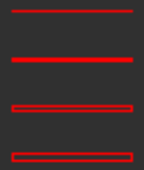Last edited:

#### emexes

##### Expert
BitmapCreator.DrawRect should draw thin lines properly.
Well, it's been an education (for me, at least). Plus it all seems pretty logical. I haven't yet gotten around to experimenting with fractional StrokeWidths, but it wouldn't surprise me to find that they too are implemented correctly. I am guessing that it took more than your customary half-hour-per-feature to program upReplies
4
Views
331
Replies
2
Views
2K
Replies
11
Views
4K
Replies
8
Views
9K
Replies
2
Views
3K